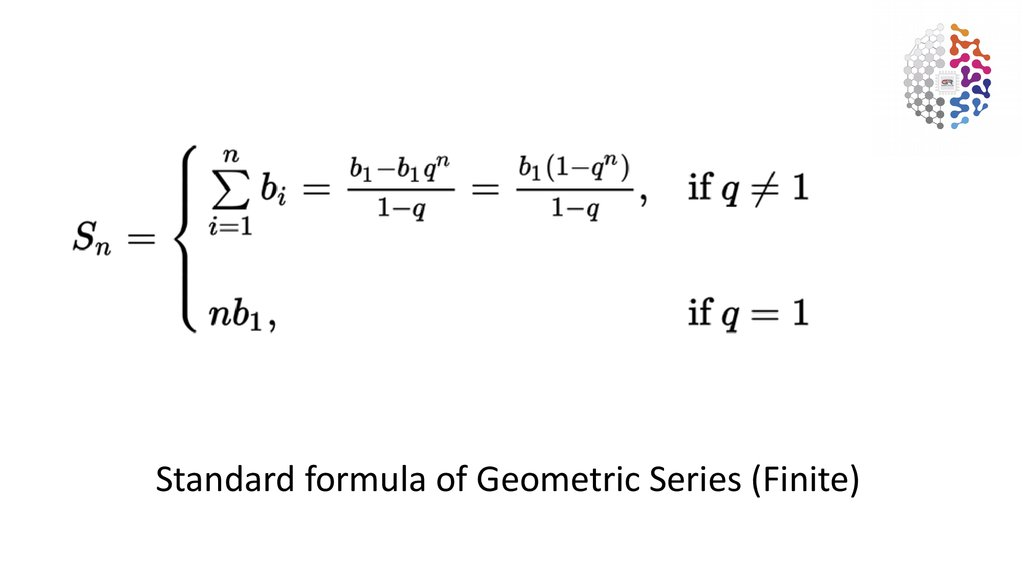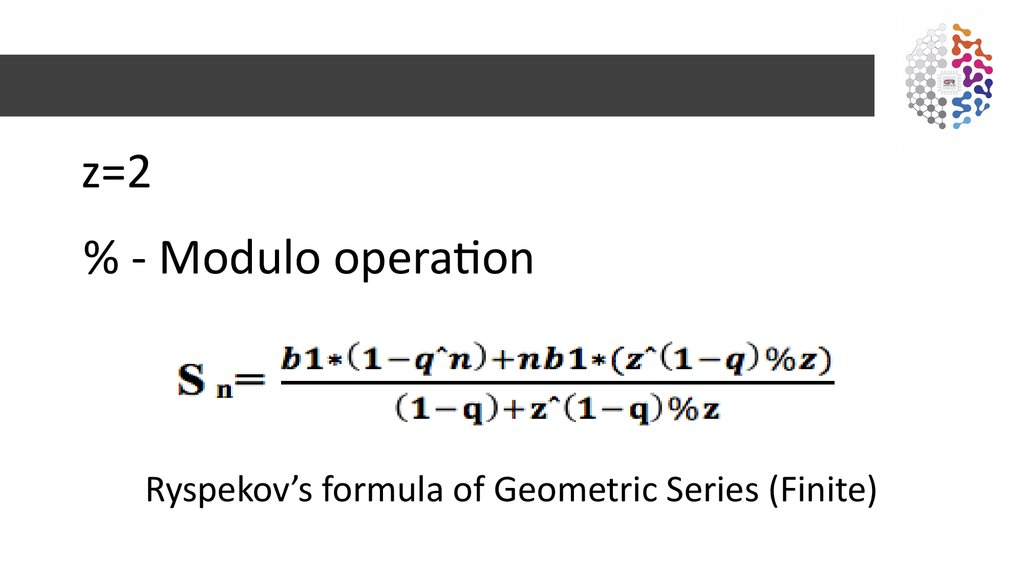# Ryspekov’s Fibonacci sequence formula Global Revival

## 2.

Standard formula of Geometric Series (Finite)

## 3.

z=2
% - Modulo operation
Ryspekov’s formula of Geometric Series (Finite)

## 4. Introduction

• Fibonacci numbers — the elements of a numerical sequence where
each subsequent number is the sum of two previous numbers. The
Fibonacci numbers are also called the Golden section. The Golden
section is used for architecture, art, space exploration, etc.
• My formula will allow easy (low resource consumption at high speed)
interact with and/or search the Fibonacci numbers (rational).
• What kind of problems with the existing formulas?

## 5. Standard formula’s problems

• Fn= Fn-1+Fn-2
• You need to know 2 or more previous numbers
So:
• High memory usage.
• If you have only one Fibonacci number, you can’t
find the next and/or previous numbers using only
this number.

max n
n=1

## 7. Binet’s formula’s problems

• Speed of search first n numbers is very slow
because the formula have a lot of operations.
• If you have only one Fibonacci number, you
can’t find the next and/or previous numbers
using only this number.

## 8. Ryspekov’s Fibonacci sequence formula. Description.

• f(x) – (2ˆx)%2
• g(x) – math rounding function (next slide)
• n – max number/count of terms
• b=n-1
• x1=1
• % - modulo operation
• y=
• %3)*(g(x)%2)+(1-f(i%3))*(f(g(x)%2))

## 9. Math Rounding (towards zero)

Math Rounding for this (Fibonacci numbers) task:
Y= (1.68…. (using only first n numbers of Phi after point)*(10ˆn)*x
Q=(Y-(Y mod (10^n)))/(10ˆn)
g(x)=Q
Example:
2.434433 will be 2
5.99999 will be 5, and etc.
Computer can do this operation without math operations
(just convert to integer): (int)(x*(1+sqrt(5))/2)

## 10. Ryspekov’s Fibonacci sequence formula.

b
i=2
All descriptions on slide 10